Courses

# Short & Long Answer Question: Equilibrium- 2 Notes | EduRev

## JEE : Short & Long Answer Question: Equilibrium- 2 Notes | EduRev

The document Short & Long Answer Question: Equilibrium- 2 Notes | EduRev is a part of the JEE Course Chemistry Class 11.
All you need of JEE at this link: JEE

Q.51 Will the pH of water be same at 4°C and 25°C? Explain.

Answer: No, pH of water is not same at 4°C and 25°C. This is because with increase in temperature, dissociation of H2O molecules increases. Hence, [H+] will increase, i.e., pH decreases. Thus, pH at 4°C will be more than at 25°C.
Q.52 The pKln of an indicator is 10.5. For which pH transition range is the indicator most suitable?

Q.53 Benzoic acid is a monobasic acid. When 1.22 g of its pure sample are dissolved in water and titrated against base, 50 ml of 0.2 M NaOH are used up. Calculate the molar mass of benzoic acid.

Answer: 1000 ml of 1 M NaOH will neutralize acid =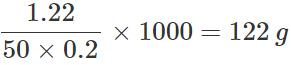1000 ml of 1 M NaOH contain 1 mole of NaOH and will neutralize 1 mole of monobasic acid. Hence, molar mass of benzoic acid =122 g mol−1.
Q.54 A solution gives yellow colour with methyl orange, methyl red and phenol red. What is the pH of the solution?

Answer: Yellow colour with methyl orange means pH > 4.5 Yellow colour with methyl red means pH > 6.2 Yellow colour with phenol red means pH < 6.4. Hence, the solution has pH between 6.2 to 6.4.
Q.55 What is the difference between ionic product and solubility product?

Answer: Solubility product is the product of the molar concentrations of the ions in a saturated solution but ionic product is for any solution.
Q.56 NaCI solution is added to a saturated solution of PbCl2. What will happen to the concentration of Pb2+ions?

Answer: Pb2+ ion concentration will decrease to keep Ksp constant.
Q.57 When is a precipitate formed when solutions of BaCl2 and Na2SO4 are mixed?

Answer: A precipitate is formed when in the final solution after mixing, the ionic product [Ba2+][SO2−4]>Ksp for BaSO4.
Q.58 Will AgCl be more soluble in aqueous solution or NaCl solution and why?

Answer: AgCl ⇌ Ag++Cl− In NaCl solution, [Cl] will increase. As [Ag+][Cl]=Ksp remains constant, [Ag+] will decrease, i.e., the solubility will be less in NaCl solution than in water.
Q.59 Why solid NaCl starts separating out from a saturated solution of NaCl if HCl gas is passed through it?

Answer: HCl in the solution provides Cl ions. This increases the ionic product of NaCl and so the solid NaCl starts separating out.
Q.60 Why common salt is added to precipitate out soap from the solution during its manufacture?

Answer: Soap is sodium salt of higher fatty acids (RCOONa). On adding common salt, Na+ ion concentration increases. Hence, the equilibrium RCOONa(s)⇌RCOO+Na+ shifts in the backward direction, i.e., soap precipitates out.
Q.61 Through a solution containing Cu2+ and Ni2+,H2S gas is passed after adding dil HCl, which will precipitate out and why?

Answer: Cu2+ will precipitate out because in the acidic medium, only ionic product [Cu2+][S2−] exceeds the solubility product of CuS.
Q.62 Why in Group V of qualitative analysis, sufficient NH4OH solution should be added before adding(NH4)2CO3 solution?

Answer: This is done to convert NH4HCO3 usually present in large amounts along with (NH4)2CO3 to (NH4)2CO3
NH4HCO3+NH4OH→(NH4)2CO3+H2O
Q.63 Two sparingly soluble salts AB and XY2 have the same solubility product. Which salt will be more soluble? Explain.

Answer: Suppose solubility of AB=a mol L1. Then AB⇌A++B,Ksp=[A+][B]=a×a=a2a=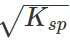Suppose solubility of salt XY2=b mol L−1. Then XY2⇌X2++2Y,Ksp=[X2+][Y]2=b(2b)2 i.e., 4b3=Ksp or b=(Ksp/4)1/3 Obviously b>a (as Ksp have values with negative powers of 10). Hence, salt XY2 is more soluble.
Q.64 The ionization constant of formic acid is 1.8×10−4. Around what pH will its mixture with sodium formate give buffer solution of highest capacity?

Answer: Buffer solution of highest capacity is formed at which pH=pKa=−log(1.8×10−4)=3.74.
Q.65 Blood is a buffer of H2CO3 and [HCO−3]with pH = 7.40. Given K1 of H2CO3=4.5×10−7. What will be the ratio of [HCO−3] to [H2CO3] in the blood?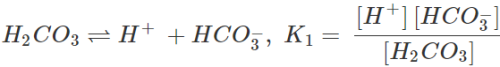or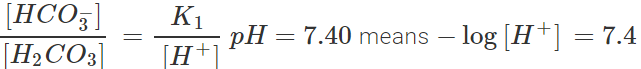or log[H+]=−7.4=8.6 or [H+]=3.981×10−8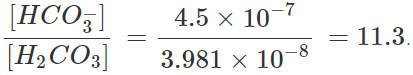Offer running on EduRev: Apply code STAYHOME200 to get INR 200 off on our premium plan EduRev Infinity!

## Chemistry Class 11

136 videos|245 docs|180 tests

,

,

,

,

,

,

,

,

,

,

,

,

,

,

,

,

,

,

,

,

,

;